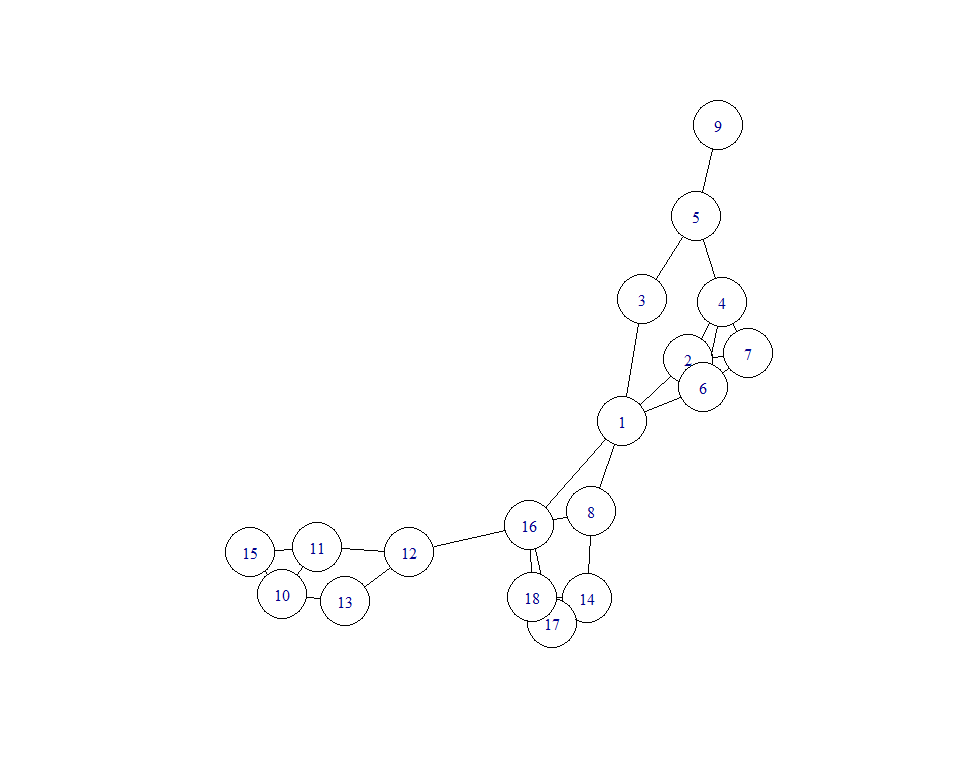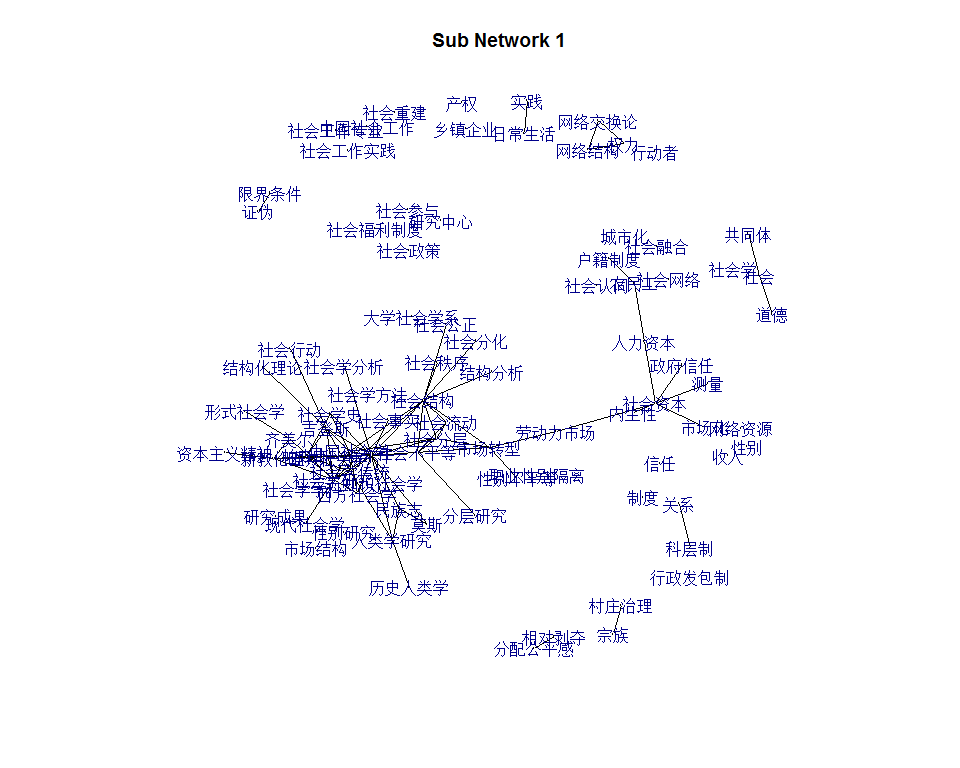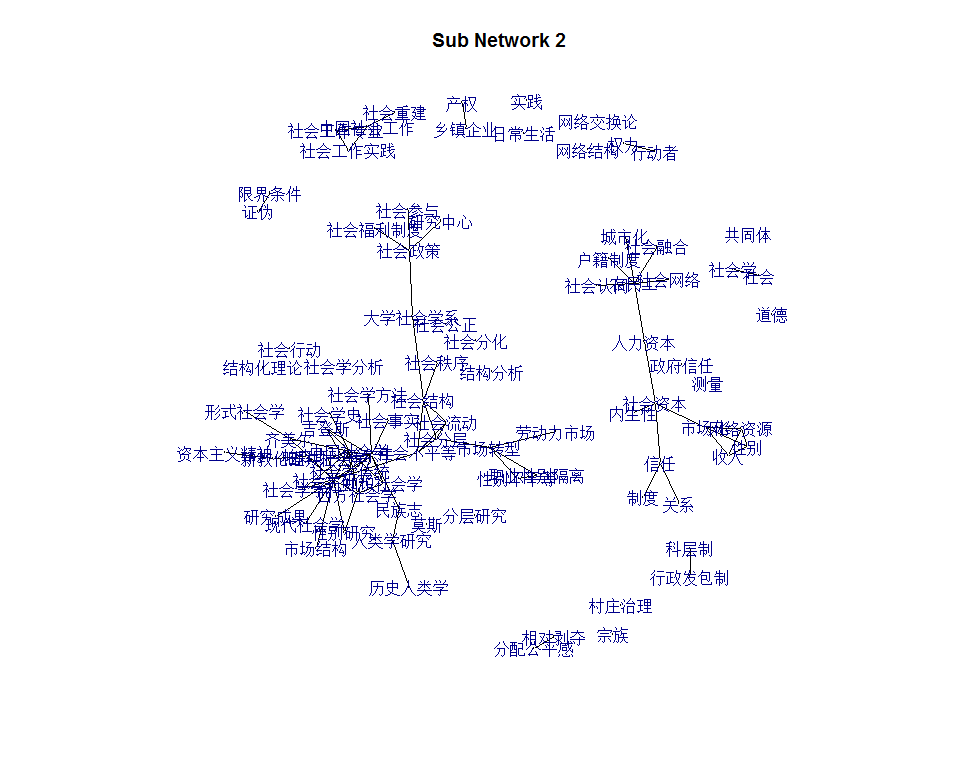# 罚似然图模型扩展

## 带协变量的罚似然图模型

$$\lambda\sum_{p = 1}^{p}\left| b_{p} \right|$$

$$\lambda\sum_{p = 1}^{m}\left| b_{p} \right|$$## 多组罚似然图模型

## 多分组罚似然图模型
## 本示例数据采用《社会学研究》和《社会》杂志2006年至2015年全部发表论文的关键词。

lapply(kw, dim)

library(JGL)
jgl.grp<- JGL(Y = kw, penalty='group', screening= 'fast', penalize.diagonal = F,
lambda1=.001, lambda2=.001)
print.jgl(jgl.grp)

theta <- jgl.grp$theta library(igraph) K=length(theta) adj = JGL:::make.adj.matrix(theta) diag(adj)=0 gadj = graph.adjacency(adj, mode="upper", weighted=TRUE) lay <- NULL if (is.null(lay)) lay <- layout.fruchterman.reingold(gadj) # class 1 class2 shared # 38 42 41 table(E(gadj)$weight)

# 公共子图
plot(gcomm, layout=lay, vertex.size=0, vertex.color = 'white',
edge.color = 'black', main = "Common Network")

# 两个子图
nets <- JGL:::make.adj.matrix(theta, separate = TRUE)
for (k in 1:K) {
g1 <- graph.adjacency(nets[[k]], mode = "undirected", diag=F)
#png(filename = sprintf('x_keywords_%d.png', k), width = 800, height = 800)
plot.igraph(g1, layout = lay, vertex.color = 'white', vertex.size = 0,
edge.color = 'black', main = paste("Sub Network", k))
#dev.off()
}